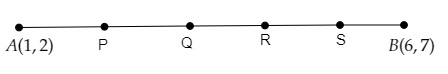# Points $P, Q, R$ and $S$ divide the line segment joining the points $A (1, 2)$ and $B (6, 7)$ in 5 equal parts. Find the coordinates of the points $P, Q$ and $R$.

Given:

Points $P, Q, R$ and $S$ divide the line segment joining the points $A (1, 2)$ and $B (6, 7)$ in 5 equal parts.

To do:

We have to find the coordinates of the points $P, Q$ and $R$.

Solution:

Let the coordinates of $P, Q, R, S$ be $(x_1,y_1), (x_2,y_2), (x_3,y_3), (x_4,y_4)$ respectively.Points $P, Q, R$ and $S$ divide the line segment joining the points $A (1, 2)$ and $B (6, 7)$ in 5 equal parts.

This implies,

$AP = PQ = QR = RS = SB$

Using the section formula, if a point $( x,\ y)$ divides the line joining the points $( x_1,\ y_1)$ and $( x_2,\ y_2)$ in the ratio $m:n$, then

$(x,\ y)=( \frac{mx_2+nx_1}{m+n},\ \frac{my_2+ny_1}{m+n})$

This implies,

$\mathrm{P}$ divides $\mathrm{AB}$ in the ratio $1: 4$
$(x_{1}, y_{1})=(\frac{1 \times 6+4 \times 1}{1+4}, \frac{1 \times 7+4 \times 2}{1+4})$

$=( \frac{6+4}{5}, \frac{7+8}{5})$

$=(\frac{10}{5}, \frac{15}{5})$

$=(2, 3)$
The coordinates of $\mathrm{P}$ are $(2,3)$.

$\mathrm{Q}$ divides $A B$ in the ratio $2: 3$.
$(x_{2}, y_{2})=(\frac{2 \times 6+3 \times 1}{2+3}, \frac{2 \times 7+3 \times 2}{2+3})$

$=(\frac{12+3}{5}, \frac{14+6}{5})$

$=( \frac{15}{5}, \frac{20}{5})$

$=(3, 4)$
The coordinates of $\mathrm{Q}$ are $(3,4)$.

$\mathrm{R}$ divides $\mathrm{AB}$ in the ratio $3: 2$.
$(x_{3}, y_{3})=(\frac{3 \times 6+2 \times 1}{3+2}, \frac{3 \times 7+2 \times 2}{3+2})$

$=(\frac{18+2}{5}, \frac{21+4}{5})$

$=(\frac{20}{5}, \frac{25}{5})$

$=(4, 5)$

The coordinates of $\mathrm{R}$ are $(4,5)$.

$\mathrm{S}$ divides $\mathrm{AB}$ in the ratio $4: 1$.
$(x_{4}, y_{4})=(\frac{4 \times 6+1 \times 1}{4+1}, \frac{4 \times 7+1 \times 2}{4+1})$

$=(\frac{24+1}{5}, \frac{28+2}{5})$

$=(\frac{25}{5}, \frac{30}{5})$

$=(5, 6)$

The coordinates of $\mathrm{S}$ are $(5,6)$.

The coordinates of the points $P, Q$ and $R$ are $(2,3), (3,4)$ and $(4,5)$ respectively.

Updated on: 10-Oct-2022

49 Views